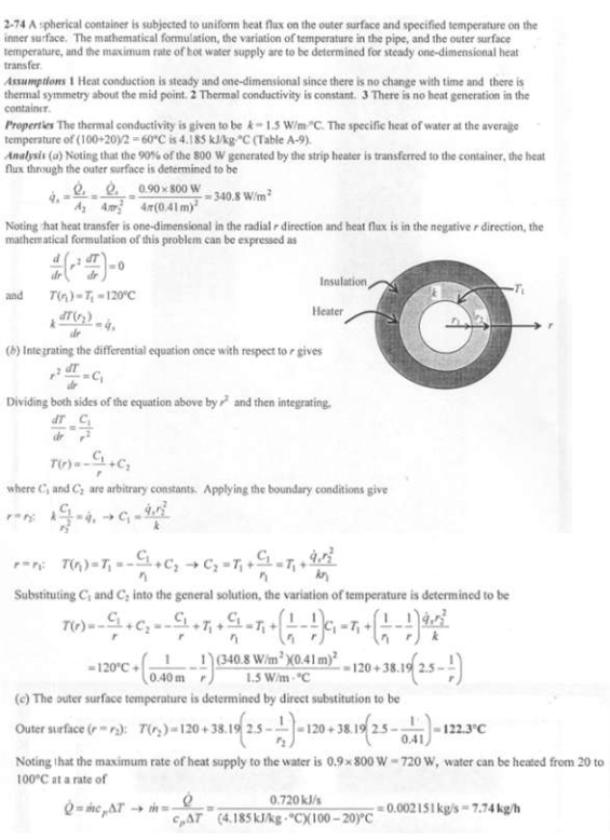heat and mass transfer problems engineering equations heat and mass transfer school homework engineering heat and mass transfer formulas heat and mass transfer solutions to heat and mass transfer problems full solution engineering problem solution heat and mass transfer math problems engineering equations heat and mass transfer school homework engineering solutions to heat and mass transfer formulas heat problems mass problem solutions to transfer problems full solution heat and mass transfer
heat and mass transfer problems engineering equations heat and mass transfer school homework engineering heat and mass transfer formulas heat and mass transfer solutions to heat and mass transfer problems full solution engineering problem solution heat and mass transfer math problems engineering equations heat and mass transfer school homework engineering solutions to heat and mass transfer formulas heat problems mass problem solutions to transfer problems full solution heat and mass transfer
Highalphabet Home Page heat and mass transfer problem solutions Heat and Mass Transfer Page
In a food processing facility, a spherical container of inner radius r_1=40 cm, outer radius r_2=41 cm, and thermal conductivity k=1.5 W/mK is used to store hot water and to keep it at 100 C at all times. To accomplish this, the outer surface of the container is wrapped with a 800-W electric strip heater and then insulated. The temperature of the inner surface of the container is observed to be nearly 120 C at all times. Assuming 10 percent of the heat generated in the heater is lost through the insulation, (a) express the differential equation and the boundary conditions for steady one-dimensional heat conduction through the container, (b) obtain a relation for the variation of temperature in the container material by solving the differential equation, and (c) evaluate the outer surface temperature of the container. Also determine how much water at 100 C this tank can supply steadily if the cold water enters at 20 C.In a food processing facility, a spherical container of inner radius r_1=40 cm, outer radius r_2=41 cm, and thermal conductivity k=1.5 W/mK is used to store hot water and to keep it at 100 C at all times. To accomplish this, the outer surface of the container is wrapped with a 800-W electric strip heater and then insulated. The temperature of the inner surface of the container is observed to be nearly 120 C at all times. Assuming 10 percent of the heat generated in the heater is lost through the insulation, (a) express the differential equation and the boundary conditions for steady one-dimensional heat conduction through the container, (b) obtain a relation for the variation of temperature in the container material by solving the differential equation, and (c) evaluate the outer surface temperature of the container. Also determine how much water at 100 C this tank can supply steadily if the cold water enters at 20 C.